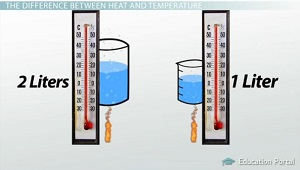# How to Calculate Specific Heat Capacity for Different Substances

An error occurred trying to load this video.

Try refreshing the page, or contact customer support.

Coming up next: Changes in Heat and Energy Diagrams

### You're on a roll. Keep up the good work!

Replay
Your next lesson will play in 10 seconds
• 0:06 How to Calculate Specific Heat
• 1:07 The Difference Between…
• 2:32 How to Calculate…
• 4:56 Application of…
• 6:11 Lesson Summary

Want to watch this again later?

Timeline
Autoplay
Autoplay
Speed

#### Recommended Lessons and Courses for You

Lesson Transcript
Instructor: John Simmons

John has taught college science courses face-to-face and online since 1994 and has a doctorate in physiology.

This lesson describes specific heat capacity and explains how the specific heat capacity of water helps to maintain a relatively constant temperature in nature. Learn how to identify the specific heat capacity of other common materials and how to calculate specific heat capacity with an experiment.

## How to Calculate Specific Heat

Have you ever wondered why it takes so long to boil water? Do you know that it takes longer to boil some liquids than it does others? For example, if you were to heat the same amount of water and alcohol, the alcohol boils first. Well, what accounts for this difference? The answer is specific heat capacity.

Specific heat capacity, or simply specific heat, is the amount of heat required to change the temperature of a substance. As water requires more time to boil than does alcohol, you might conclude that water requires more heat than alcohol to raise its temperature. In other words, water has a higher specific heat capacity. That conclusion would be accurate. To better understand specific heat capacity, we need to distinguish between heat and temperature, as both of these terms are used in its definition.

## The Difference Between Heat and Temperature

Let's look at an example to help us understand the difference between heat and temperature. Consider two beakers of boiling water with 1 liter in one and 2 liters in the other. If both are boiling, they have the same temperature. You see, temperature is defined as the average amount of kinetic energy in the substance, where kinetic energy is the energy of motion. The molecules in each pot have the same average energy of motion or average amount of kinetic energy. In other words, the water molecules in each pot are moving at the same speed. Temperature is measured in degrees Celsius, degrees Fahrenheit, or even Kelvin.However, two liters of boiling water contain more heat than one liter of boiling water, even though they have the same temperature. In the context of specific heat capacity, heat is the total amount of energy in a substance, and heat is sometimes referred to as heat energy, in which energy is the ability to do work. In the scientific community, energy is measured in joules but also can be expressed as calories.

While heat and temperature are different measures, they are certainly related. Temperature increases as heat is added to a substance. Likewise, temperature decreases as heat is removed.

## How to Calculate Specific Heat Capacity

Let's do a little experiment. All we need is a beaker of water, a thermometer, and a power supply that generates heat. The power supply will measure the amount of heat that we add to the water. Let's say that we heat 10 mL (10 grams) of water 10 degrees Celsius. In doing so, the power supply registers 420 joules. That means it took 420 joules of heat energy to raise 10 grams of water 10 degrees Celsius.

We can use this information to calculate the specific heat capacity of water. All we need is to figure out how much heat is required to raise the temperature of one gram of water one degree Celsius.

The equation for specific heat is as follows:

S.H.C. = Heat Energy / (mass of substance * change in temperature)

The equation is read 'specific heat capacity equals heat energy per gram per degree Celsius.'

Now, if we plug in our results, we get the following:

S.H.C. = 420 joules / (10 grams * 10 degrees Celsius) = 4.2 joules per gram per degree Celsius

What does that mean? That means it takes 4.2 joules of heat energy to raise one gram of water one degree Celsius. To make it even easier to remember, let's convert joules to calories. Remember, both are units for energy. There are 4.2 joules in 1 calorie. Therefore, we can say the specific heat capacity of water is 1 calorie per gram per degree Celsius. That's pretty easy to remember!

To unlock this lesson you must be a Study.com Member.

### Register to view this lesson

Are you a student or a teacher?

### Unlock Your Education

#### See for yourself why 30 million people use Study.com

##### Become a Study.com member and start learning now.
Back
What teachers are saying about Study.com

### Earning College Credit

Did you know… We have over 160 college courses that prepare you to earn credit by exam that is accepted by over 1,500 colleges and universities. You can test out of the first two years of college and save thousands off your degree. Anyone can earn credit-by-exam regardless of age or education level.